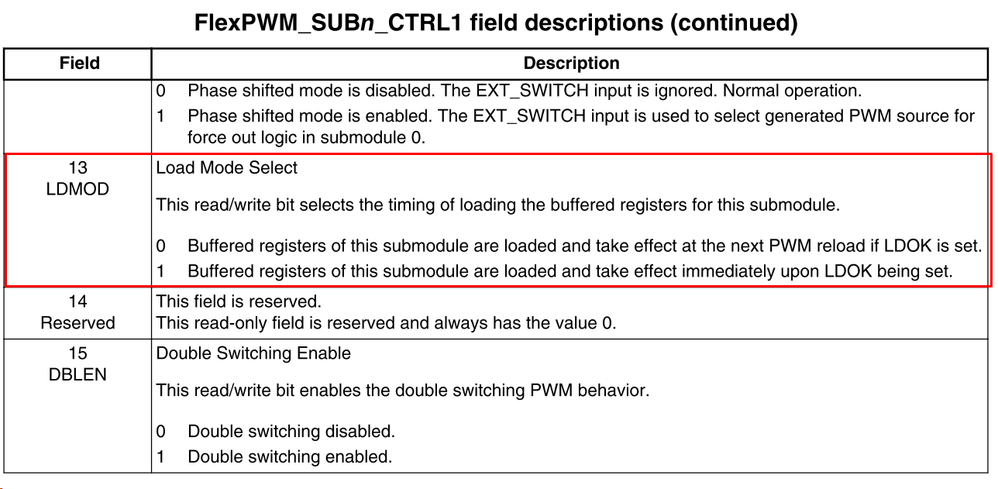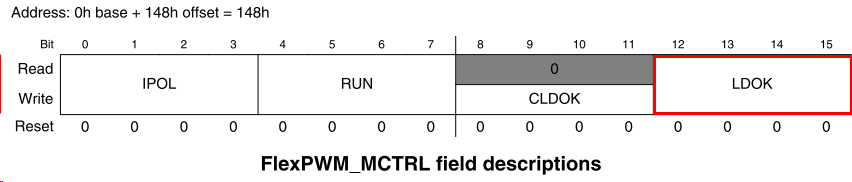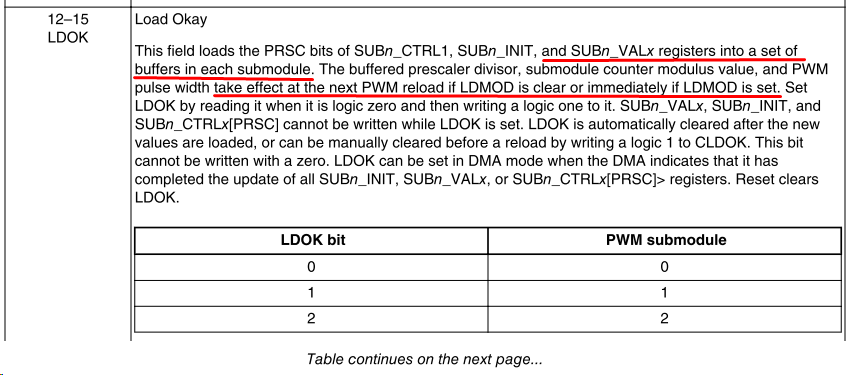# Change of PWM periode on runtime

cancel
Showing results for
Did you mean:

## Change of PWM periode on runtime

376 ViewsContributor I

Dear community,

I would like to ask, if it is possible to change the periode of PWM (frequency) on runtime?

I am using B0, B3, X0 and X3 outputs. If I try to change the FlexPWM_0.SUB.VAL5.R for example, the duty cycle of B0 output will change without problem. If I try to change FlexPWM_0.SUB.VAL4.R, nothing will be effected. What am I doing wrong?

Thank you for any help in advance.

Following is my configuration:

FlexPWM_0.OUTEN.R           = 0x0099; // b3 b0 x3 x0
//sub 0
FlexPWM_0.SUB.CTRL1.R    = 0x0404; // full cycle reload, LDMOD
FlexPWM_0.SUB.CTRL2.R    = 0x2000; // independent
FlexPWM_0.SUB.DTCNT0.R   = 0x0000; // deadtime values, not aplicable on INDEP mode
FlexPWM_0.SUB.DTCNT1.R   = 0x0000;
FlexPWM_0.SUB.INIT.R    = 0x0;  // PWM init value
FlexPWM_0.SUB.VAL0.R   = PWM_MODULO/2;   // PWMX rising edge, half cycle reload point   x0
FlexPWM_0.SUB.VAL1.R   = PWM_MODULO; // PWM modulo, PWMX falling edge
FlexPWM_0.SUB.VAL2.R   = 0;     // PWM A0 rising edge
FlexPWM_0.SUB.VAL3.R   = 0;  // PWM A0 falling edge
FlexPWM_0.SUB.VAL4.R   = PWM_MODULO/2;  // PWM B0 rising edge      b0
FlexPWM_0.SUB.VAL5.R   = PWM_MODULO; // PWM B0 falling edge
FlexPWM_0.SUB.DISMAP.R = 0x0000;  // disable fault pin condition

//sub 3
FlexPWM_0.SUB.CTRL1.R    = 0x0404; // full cycle reload, LDMOD
FlexPWM_0.SUB.CTRL2.R    = 0x2000; // independent
FlexPWM_0.SUB.DTCNT0.R   = 0x0000; // deadtime values, not aplicable on INDEP mode
FlexPWM_0.SUB.DTCNT1.R   = 0x0000;
FlexPWM_0.SUB.INIT.R    = 0x0;  // PWM init value
FlexPWM_0.SUB.VAL0.R   = PWM_MODULO/2;   // PWMX rising edge, half cycle reload point    x3
FlexPWM_0.SUB.VAL1.R   = PWM_MODULO; // PWM modulo, PWMX falling edge
FlexPWM_0.SUB.VAL2.R   = 0;     // PWM A0 rising edge
FlexPWM_0.SUB.VAL3.R   = 0;  // PWM A0 falling edge
FlexPWM_0.SUB.VAL4.R   = PWM_MODULO/2;  // PWM B0 rising edge
FlexPWM_0.SUB.VAL5.R   = PWM_MODULO; // PWM B0 falling edge                             b3
FlexPWM_0.SUB.DISMAP.R = 0x0000;  // disable fault pin condition

FlexPWM_0.MCTRL.R = 0x0900;

Tags (1)
3 Replies
251 ViewsNXP Employee

Hi,

It looks like you have not enable the reload of double buffered registers on new period (in your case imediatelly).

After you load new values to VALx register (bouble buffered) set LDOK for particular module in order to load double buffered values to VALx registers.Regards,

Peter

251 ViewsContributor I

Thank you very much for the quick reply.

Initial value for PWM_MODULO is 3548, so after initialization I have the PWM period as 0.222 ms and the duty cycle is 50%, which is perfect. Then I tried in my test routine with g_ustest_i as 2346, only the duty cycle (about 32.4%) is changed not the period. I have expected that I get the period changed to 0.147ms and the duty cycle unchanged (50%).

I have checked the SFR registers. The values should have been correctly written.

Test routine:

if (g_ustest_j)

g_ustest_j=0;
FlexPWM_0.SUB.VAL4.R = g_ustest_i/2;    //b0
FlexPWM_0.SUB.VAL5.R = g_ustest_i;
if (!FlexPWM_0.MCTRL.B.LDOK)
FlexPWM_0.MCTRL.B.LDOK = 0x1;  //write 1 to load the values
while (FlexPWM_0.MCTRL.B.LDOK == 0x1);
}

251 ViewsContributor I

An update for my progress. I tried to change the VALs of all used outputs as follows, in my case B0 and X0 of submodule 0, then it works.

if (g_ustest_j)

g_ustest_j=0;
FlexPWM_0.SUB.VAL0.R = g_ustest_i/2;   // PWMX rising edge, half cycle reload point   x0
FlexPWM_0.SUB.VAL1.R = g_ustest_i;

FlexPWM_0.SUB.VAL4.R = g_ustest_i/2;    //b0
FlexPWM_0.SUB.VAL5.R = g_ustest_i;
if (!FlexPWM_0.MCTRL.B.LDOK)
FlexPWM_0.MCTRL.B.LDOK = 0x1;  //write 1 to load the values
while (FlexPWM_0.MCTRL.B.LDOK == 0x1);
}

At this point I would have another question, is it possible to have 2 outputs in one submodule, in my case B0 and X0, fix shifted? I have only seen some examples with shifted PWM in different submodules.

___                     ___

B0: __|     |__________|     |___________

___                     ___

X0: _____|     |__________|     |___________